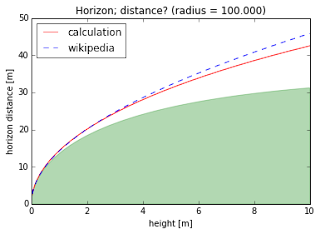## What is the Horizon?

### Introduction

When writing the 200 reasons blogpost, one of the things I noted and remarked upon is that flat earthers do not seem to know what the Horizon actually is.

This post is dedicated to provide that information. This also means it is slightly mathematical, although it does not require much beyond high school level math.

### The Horizon: Where is it?

So this is the first derivation. The concept is pretty simple, although somehow it has not been made clear to a large number of people.

Draw a circle. Now, pick a point - any point outside the circle will do. Now, grab a ruler and make lots of lines. What do you find? There are, in essence, three types of lines. First, there are lines that aren't coming near the circle. Second, there are lines that intersect the circle - passing through it twice. And there are those very special lines that only intersect once. You'll also find that, from any given point, there are only two of these very special lines.

Now, imagine taking that circle and rotating it about the line through its center and the point you picked. The both of us know that you'll end up with a sphere. If you do the same two the two lines, then instead of two points you'll have a circle on the sphere's surface. The circle is centered on the point (or rather, the axis) and therefore the points on the circle are equidistant from its center.

That's the Horizon.

What people don't realise is that we can actually derive this. Let's do that!

The equation for a circle of radius $r$ in cartesian $(x,y,z)$ coordinates is: \begin{align} x^2 + y^2 &= r^2\end{align}

The solution to that is two lines, namely $y=\pm \sqrt{r^2-x^2}$. These are just the upper and lower half of the sphere, so let's ignore that.

The equation for a line is a lot simpler:  \begin{align}y&=y_0 + a\:x\end{align} That'd be a line through the point $(0, y_0)$, or equivalently intersecting the vertical axis at height $y_0$. Let's put your eyes up there. If the circle has radius $r$, your eyes are at $y_0 = r + h$, where $h$ represents your eye level.

This gives us two equations with two unknowns, namely $x$ and $y$. This is solvable:
\begin{align} x^2 + (y_0 + a x)^2 &= r^2 \\ x^2 + y_0^2 + a^2x^2 + 2 a y_0 x - r^2 &= 0 \\ (1+a^2) x^2 + (2 a y_0 ) x + (y_0^2-r^2) &= 0 \end{align}

I know, math is scary. The thing is, this is math from the second-year of high school, quadric equations. We want to look at the case where there is only one solution to this equation, which requires that the determinant is zero. In other words, if we write $\alpha x^2 + \beta x + \gamma = 0$, we require that $\beta^2=4\alpha\gamma$.I wrote a small bit of code on DataJoy to show what we've been doing. The two green lines are the final result of ourcalculation. As you can see, they touch the sphere - only oneintersection.
\begin{align}4a^2y_0^2&=4(1+a^2)(y_0^2-r^2)\\a^2(y_0^2-y_0^2+r^2) &= (y_0^2-r^2)\\a^2 &= \frac{y_0^2}{r^2}-1\\a&=\pm\sqrt{ \frac{y_0^2}{r^2}-1}\end{align}

So, there are two values of $a$ satisfying this property. This is not surprising - after all, a circle is symmetric in the vertical axis! Indeed, these two lines are the equivalent of turning around to look at the other horizon.

Our solution can easily be visualised, so I did.

### How far is the Horizon?

This is an interesting question. We need to know the distance between two points on a circle; this is easy, because it is the radius times the angle between the two points and the center of the sphere.

We can calculate that. Since we've determined $a$, we can determine the coordinates of the intersection, which was $x = \frac{-\beta}{2\alpha}$.

\begin{align}x_\text{intersection}&=\frac{-\beta}{2\alpha}\\&= - \frac{a y_0}{1 + a^2}\\y_\text{intersection} &= y_0 + a x_\text{intersection}\end{align}

The angle is then $\theta = \text{arctan}\left( \frac{y_\text{intersection}}{x_\text{intersection}}\right)$. The angular difference, since our point is on the vertical axis ($\phi=\frac{\pi}{2}$ in radians, or 90 degrees), we need $\Delta \phi= \frac{\pi}{2}-\theta$.I wrote another bit of code, this time to show you thedistance to the horizon. In Green, we see the calculationoutlined in the text. In red, we calculate the line-of-sightdistance, which we compare to wikipedia's formula (from itssource, without approximations).
That, finally, gives us the distance. Instead of going through the formulas again, I plotted it. Now, one remark. The derivation we did contains no approximations, and defines distance over the sphere. This means that if you see a city in the distance, and drive to it, then our formula gives you the distance you drive. However, this is not the same as the distance between you and the object - which is a straight line, while driving over the sphere is a curved line.

This does however give us a wonderful opportunity to see curvature in action. For a relatively small sphere, we find the image to the right. As you can see, there's a small difference between our result and wikipedia's source. However, I'm calling that on a numerical error rather than a analytic disagreement.

How would it look if we enter Earth's radius? That's what we are interested in, right?

That looks good. We see that our calculation and wikipedia now agree fully, and the line of sight versus curved distance are the same. Or rather, the height is now so small that those two don't know the difference.

That concludes our calculation.

### Summary

We've seen how to use high school mathematics (geometry) to derive the distance of the horizon. Hopefully, a number of people are now able to convincingly explain what the horizon is without silly misunderstandings.

Such as "The horizon is always on eye-level!" - that's right, because that's the green line in the first plot.